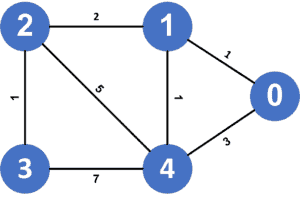If you have a few years of experience in Computer Science or research, and you’re interested in sharing that experience with the community, have a look at our Contribution Guidelines.

## 1. Overview

In this tutorial, we’ll discuss finding the shortest path in a graph visiting all nodes.

First, we’ll define the problem and provide an example that explains it. Then, we’ll discuss two different approaches to solve this problem.

## 2. Defining the Problem

Suppose we have a graph,, that containsnodes numbered fromto. In addition, we haveedges that connect these nodes. Each of these edges has a weight associated with it, representing the cost to use this edge.

Our task is to find the shortest path that goes through all nodes in the given graph.

Recall that the shortest path between two nodesandis the path that has the minimum cost among all possible paths betweenand.

In this task, we look for all the paths that cover all possible starting and ending nodes. Besides, we can visit the same node more than once. Let’s look at an example for better understanding.

Suppose we have the following graph:As we can see, there’re many paths that go through all nodes in the given graph, but the shortest path among them, and it has a cost equal to; therefore, the shortest path in the given graph visiting all nodes has a cost equal to.

## 3. Brute Force Approach

### 3.1. Main Idea

The main idea here is to generate all the possible paths and get the one that has the minimum cost.

Firstly, we’ll generate all the possible permutations of nodes. Each permutation will represent the order of visiting nodes in the graph. A path’s cost will be equal to the sum of all shortest paths between every two consecutive nodes.

Secondly, we’ll calculate the shortest path between every two consecutive nodes using the Floyd-Warshall algorithm. Recall that the Floyd-Warshall algorithm calculates the shortest path between all pairs of nodes inside a graph.

Finally, the shortest path visiting all nodes in a graph will have the minimum cost among all possible paths.

### 3.2. Implementation

Let’s take a look at the implementation:Initially, we declare an array called, which stores the shortest path between every pair of nodes in the given graph using the Floyd-Warshall algorithm.

Next, we generate all the possible permutation which represent all the possible paths we could follow. Then for each permutation, we’ll calculate the cost of it by adding the length of the shortest path between every two consecutive nodes; after calculating the cost of the current permutation, we’ll check if theis less than thevalue, then we’ll update the.

Finally, we return, which stores the cost of the shortest path visiting all nodes in the given graph.

### 3.3. Complexity

The time complexity here is. Let’s examine the reason behind this complexity.

First, the Floyd-Warshall algorithm has complexity. Next, the number of all different permutations ofnodes is equal to the factorial of, and for each permutation, we calculate its cost by passing through it, so it’s.

Therefore, the total complexity will be.

## 4. Dijkstra Approach

### 4.1. Main Idea

In this approach, we’ll use a modified version of the Dijkstra algorithm. For each state, we need to keep track of all the visited nodes besides the current one. So, to get all the visited nodes in each state, we’ll represent them as bitmask where each bit that’s turned on means that we’ve visited this node before.

In the end, our answer we’ll be the minimum value among all nodes’ values that have a bitmask full of ones (visited all nodes).

### 4.2. Implementation

Let’s take a look at the implementation:Initially, we declare an array called, which stores the shortest path to some node visiting a subset of nodes. We also declare a priority queue that stores a node and a bitmask. The bitmask represents all visited nodes to get to this node. This priority queue will sort states in acceding order according to the cost of the state.

Next, we try to start the shortest path from each node by adding each node to the priority queue and turning their bit on. Then, we run the Dijkstra algorithm.

We iterate over the children nodes of thenode. For each, we check if thevalue of it visiting a specific subset of nodes greater than the currentplus the weight of the edge that connects thenode with the. In this case, we need to update thevalue of thenode. Also, we add theand the currentbitwise-orto the priority queue. This mask means that we turn the bit of thenode on.

Finally, our answer will be the minimum value among all the nodes cost visiting all nodes.

### 4.3. Complexity

The time complexity here is, whereis the number of nodes andis the number of all possible subset of nodes, andfor adding each state to the priority queue.

## 5. Conclusion

In this article, we presented the problem of finding the shortest path visiting all nodes. We explained the main ideas behind two different approaches to solving this problem and walked through their implementation.

If you have a few years of experience in Computer Science or research, and you’re interested in sharing that experience with the community, have a look at our Contribution Guidelines.